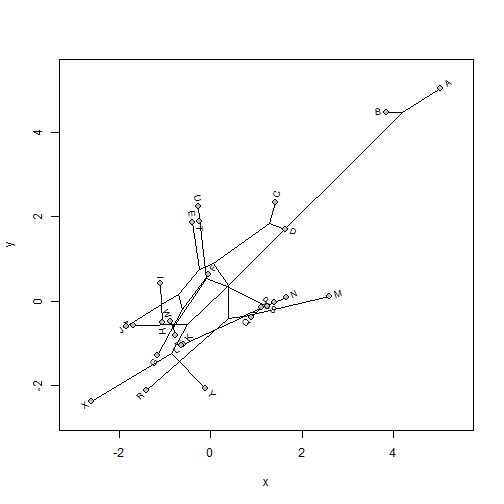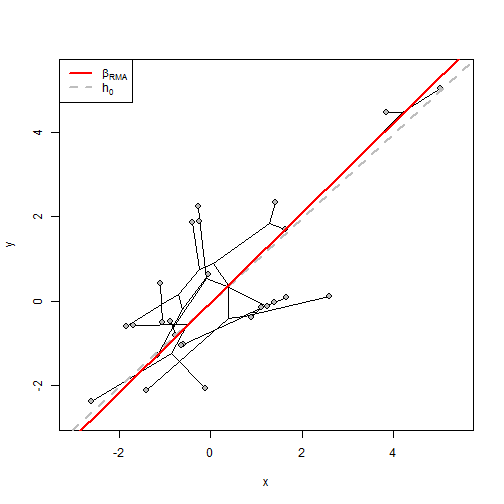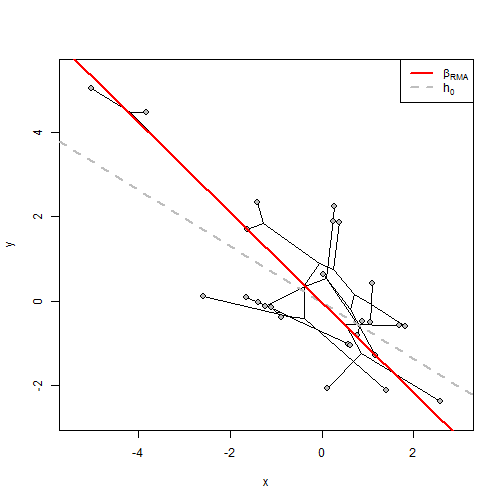Thursday, December 29, 2016

Methods & object class for phyl.RMA (phylogenetic RMA regression)

I just added S3 print, residuals, and coef methods for the phylogenetic reduced major axis regression function, phyl.RMA. The updates can be seen here.

The following is a quick demo using simulated data:

library(phytools)
packageVersion("phytools")
##  '0.5.68'
tree
##
## Phylogenetic tree with 26 tips and 25 internal nodes.
##
## Tip labels:
##  A, B, C, D, E, F, ...
##
## Rooted; includes branch lengths.
x
##           A           B           C           D           E           F
##  5.02625173  3.84069323  1.40907359  1.62584177 -0.38820852 -0.03660051
##           G           H           I           J           K           L
## -1.15405529 -1.05555766 -1.10500369 -1.82982215 -0.58220778 -0.62621299
##           M           N           O           P           Q           R
##  2.58984857  1.65864863  1.38732527  1.10492094  0.89527230 -1.40154533
##           S           T           U           V           W           X
##  1.23500803 -0.24029572 -0.26507837 -0.77611134 -0.88118355 -2.59262521
##           Y           Z
## -0.11687224 -1.68772212
y
##           A           B           C           D           E           F
##  5.03364484  4.45525372  2.33034028  1.70260435  1.86376515  0.63005315
##           G           H           I           J           K           L
## -1.27847189 -0.51986100  0.42627759 -0.59603022 -1.02732412 -1.06377260
##           M           N           O           P           Q           R
##  0.11285994  0.08066473 -0.02928941 -0.14296226 -0.38034287 -2.11329071
##           S           T           U           V           W           X
## -0.12823996  1.88409506  2.23634682 -0.82779628 -0.49460318 -2.37103424
##           Y           Z
## -2.07654579 -0.58623675
phylomorphospace(tree,cbind(x,y),node.size=c(0,0))
points(cbind(x,y),cex=1.2,pch=21,bg="grey")Now let's run the RMA regression:

obj<-phyl.RMA(x,y,tree)
## print
obj
##
## Coefficients:
## (Intercept)           x
##  -0.0424625   1.0647447
##
## VCV matrix:
##          x        y
## x 1.504905 1.119709
## y 1.119709 1.706083
##
## Model for the covariance structure of the error is "BM"
##
## Estimates (or set values):
##    lambda    log(L)
##   1.00000 -69.49404
##
## Hypothesis test based on Clarke (1980; Biometrika):
##        r2         T        df         P
##  0.488316  0.429650 21.290154  0.671767
##
## Note that the null hypothesis test is h0 = 1
coef(obj)
## (Intercept)           x
##  -0.0424625   1.0647447
residuals(obj)
##            A            B            C            D            E
## -0.275567308  0.408358649  0.872499213  0.013960519  2.319570602
##            F            G            H            I            J
##  0.711485847 -0.007235186  0.646500875  1.645286860  1.394725642
##            K            L            M            N            O
## -0.364958989 -0.354553159 -2.602204971 -1.642910026 -1.463974066
##            P            Q            R            S            T
## -1.276958414 -1.291116753 -0.578540317 -1.400745657  2.182411146
##            U            V            W            X            Y
##  2.561050105  0.041026621  0.486094798  0.431912090 -1.909644200
##            Z
##  1.253218854

I also wrote a simple plot method that throws the RMA line on top of a plotted phylomorphospace. Details can be seen here.

This is what it looks like:

plot(obj)We can also flip x or y just to see what it looks like to plot a negative slope. Note that we can only test a null hypothesis that has the same sign as our fitted RMA line. Here (for fun), I'll test the null hypothesis h0 = -2/3.

neg.x<--x
obj<-phyl.RMA(neg.x,y,tree,h0=-2/3)
obj
##
## Coefficients:
## (Intercept)           x
##  -0.0424625  -1.0647447
##
## VCV matrix:
##           x         y
## x  1.504905 -1.119709
## y -1.119709  1.706083
##
## Model for the covariance structure of the error is "BM"
##
## Estimates (or set values):
##    lambda    log(L)
##   1.00000 -69.49404
##
## Hypothesis test based on Clarke (1980; Biometrika):
##        r2         T        df         P
##  0.488316  3.206537 21.290154  0.004187
##
## Note that the null hypothesis test is h0 = -0.666666666666667
plot(obj)That's the idea anyway.

Data for this demo were simulated as follows:

library(phytools)
tree<-pbtree(n=26,tip.label=LETTERS)
xy<-fastBM(tree)
x<-xy+fastBM(tree,sig2=0.4)
y<-xy+fastBM(tree,sig2=0.4)